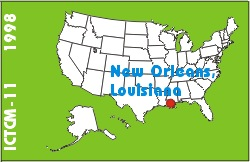# A graphical tool for analysis courses

### Jeff L. Hirst

Department of Mathematical Sciences
Appalachian State University
Boone, NC 28608
USA
Phone: (828) 262-2861

list of all papers by this author

 Click to access this paper: paper.html

## ABSTRACT

Due to the reform movement, many universities use technology in their calculus courses. By using computer algebra systems in higher level courses, we can capitalize on skills students are learning in their introductory courses. This paper describes a Maple procedure that illustrates the mechanics of delta-epsilon proofs.

The Maple procedure works as follows. Students enter a function, a point in the domain of the function, an expected limit, and the maximum value of epsilon to be considered. The procedure then generates an animation where each frame corresponds to a value of epsilon. Each frame displays the graph of the function, highlighting the region of the range corresponding to epsilon and the region of the domain corresponding to delta. Additionally, a graph of the delta values associated with each value of epsilon is provided. Students can apply modeling skills to find a formula for delta in terms of epsilon.

Besides providing the Maple code for the procedure and some sample frames from the output, this paper includes some tips on using the display command in Maple and on distributing Maple code in the classroom.

Keyword(s): calculus, limits, Maple